Incredibly Helpful GMAT Tutor NYC & Online

# If n is a positive integer and n^2 is divisible by 72, then the largest positive integer that must divide n is GMAT Explanation, Video Solution, and More Practice!

If n is a positive integer and n^2 is divisible by 72, then the largest positive integer that must divide n is

A. 6
B. 12
C. 24
D. 36
E. 48

We get this one a lot in GMAT tutoring sessions. Most people fail to understand what the questions is asking and then just start working without a plan. It ends up being that work itself isn’t totally off but the student doesn’t really know where to take it in order to narrow down the answer choices.

What also tends to confuse is that the question asks for the largest positive integer but we end up choosing the smallest possible value of n.

## Define the question

the largest positive integer that must divide n

There it is. But let’s not leave it like that. Always try to do something with the questions. In this case because we’re looking for something that divides evenly into n let’s set up a fraction:

n/z = integer

And we want to maximize z because we’re looking for the largest integer that must divide n.

The largest integer that divides any integer is itself. So really we’re looking for n. Not that important to make that inference but just wanted to point it out.

## Setup

Now let’s gather the information from the question and get things set up so we can make some inferences. We know that n is a positive integer and then n squared is divisible by 72. We can write out an equation with that second piece of information.

n^2/72 = integer

We’re really looking to solve for n so let’s go ahead and simplify this equation.

n^2 = integer*72

Take the square root of both sides.

n = √72√integer

Now let’s pull out perfect squares from 72.

n = √9√4√2√integer

n = 6√2√integer

Now we can use the first piece of information that n is a positive integer. So 6√2√integer is a positive integer. Somehow the radicals have to disappear. So √2•√integer must be an integer. What’s an easy way to do that? Make integer equal 2 so you have √2•√2 = 2.

n = 6*2 = 12

What’s the largest positive integer that must divide n? 12

Now, you might be thinking: is 12 the only possibility for n? Or put another way, is 2 the only possible value for the integer? Good question. No. There are an infinite number of possible values for the integer and consequently for n. Any number that cancels out the radicals will work.

√2√8 = √2√2√4 = 4*6 = 24

√2√18 = √2√2√9 = 6*6 = 36

√2√32 = √2√2√16 = 8*6 = 48

Any number that has √2•perfect square will work.

So why is the integer 2 and the correct n 12? This is coming back to what tends to confuse GMAT tutoring students (looking for the largest integer that must divide but then the answer is actually the smallest possible value of n).

Because the question is a MUST. So you need the most basic building block of n. Look at all of the values we came up with for n: 12, 24, 36, 48. What’s the biggest integer they have in common (least common multiple)? 12

Regardless of which multiple of n you come up with it will always be divisible by 12.

## Additional GMAT Divisibility Practice Question

Here’s another divisibility question from GMAT Official Guide: If x and y are positive integers such that y is a multiple of 5 and 3x + 4y = 200, then x must be a multiple of which of the following?

Here’s a tricky exponents divisibility puzzle from GMAT question of the day

And another from the GMAT official guide that’s not the same but has a similar puzzle/exponents/divisibility vibe with factorials in the mix: If p is the product of the integers from 1 to 30, inclusive, what is the greatest integer k for which 3^k is a factor of p?

# Last year the price per share of Stock X increased by k percent and the earnings per share of Stock X increased by m percent, where k is greater than m. By what percent did the ratio of price per share to earnings per share increase, in terms of k and m? GMAT Explanation, Video Solution, and More Practice!

Last year the price per share of Stock X increased by k percent and the earnings per share of Stock X increased by m percent, where k is greater than m. By what percent did the ratio of price per share to earnings per share increase, in terms of k and m?

A. k/m
B. (k–m)
C. 100(k–m)/(100+k)
D. 100(k–m)/(100+m)
E. 100(k–m)/(100+k+m)

This is a very challenging percent change word problem with a ton of variables from the GMAT Official Guide. A few things to remember when working on word problems:

2. Define the question before starting calculations (use the nouns to define the question you don’t have to fill in the numbers)
3. Solve thoughtfully. Avoid simply burrowing in. Zoom in/Zoom out. Pause. Think.

Since there are variables in the answer choices this is a good candidate for: picking numbers.

Now, let’s also remember the practical picking numbers test. If the question would be easier if you simply had the numbers then go ahead and pick them! This question would just be a percent change. So I’d say that is a clear signal to pick numbers.

Alright, we’re getting a little ahead of ourselves. Let’s go ahead and define the question: By what percent did the ratio of price per share to earnings per share increase, in terms of k and m?

That’s it, no more no less. In tutoring sessions students often struggle getting this question defined. The question is always just that last line and doesn’t need interpretation. If you’re having trouble defining it just read the last line to yourself word by word verbatim. Often, if you’re confused it’s because you missed a word or added a word or two. Avoid paraphrasing here.

What gets missed on this one? That you’re comparing RATIOS. It’s the percent change of the RATIO of price per share to earnings per share. It’s not Price compared to Earnings. It’s P/E last year compared to P/E now.

So let’s put that information into the percent change formula:

(((New Price Per Share/New Earnings Per Share)/(Old Price Per Share/Old Earnings Per Share)) – 1)100. The general formula is ((New/Old) – 1)100

We have a bunch of variables. The New/Old price and earnings and k and m. Are there any constraints? k > m. But that’s it. So you can pretty much pick whatever numbers you’d like.

Any thoughts on where to start? I’d start by picking the old price and earnings then k and m which we’ll use to derive the new price/earnings.

Because we’re dealing with percents let’s use 100 because that makes things easy.

Can you make earning and price the same? Yes! Why not?

Old

Price 100

Earnings 100

Now let’s pick an easy k and m. I’d go for 10 and 5. or 20 and 10. Something simple. Let’s do 10 and 5.

New

Price 110

Earnings 105

Now let’s put those numbers into our percent change formula.

((110/105)/(100)(100) – 1)

Notice that by making old price and earnings the same the denominator cancels. So after cancelling the denominator and reducing 110/105 we’re left with:

(22/21 – 1 )100 = (22/21 – 21/21)100 = 100/21

So that’s our percent change, 100/21. Now we need to do what we always do picking numbers: plug the numbers into the answer choices to yield our answer, 100/21.

If you follow our blog and have read our other GMAT explanations you probably know that we have a shortcut for this. We’re going to use divisibility to avoid some of the calculations.

Let’s look at the denominator of our answer: 21. So we know that the correct answer must have a denominator that is a multiple of 21. I’d break down 21 to primes, 7 and 3. I’d focus on three because it has easy divisibility rules. So we also know that the denominator must be a multiple of 3. So let’s start eliminating answer choices based on that.

A. k/m

10/5 = 2. Not Div by 3.

B. (k–m)

10-5 = 5. Not Div by 3.

C. 100(k–m)/(100+k)

110. Not Div by 3.

D. 100(k–m)/(100+m)

105 is Div by 3 so this is possible.

E. 100(k–m)/(100+k+m)

115 is not Div by 3.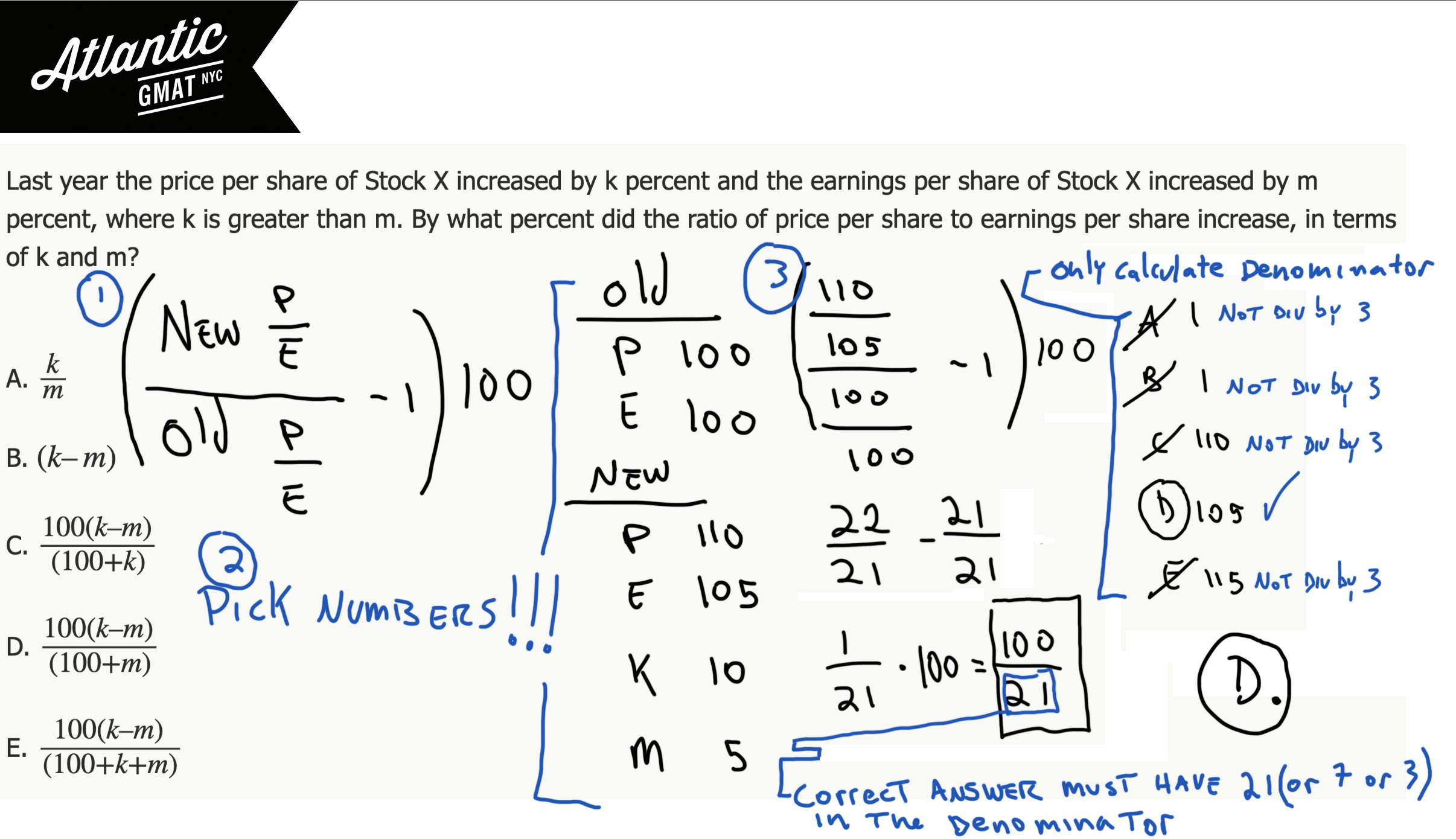## More Challenging GMAT Word Problem Practice Questions

Here’s an almost identical question from the GMAT Official Guide: Last Sunday a certain store sold copies of Newspaper A

Here’s a work and rate example that’s a little different but you try picking numbers and using the divisibility trick for eliminating answers: During a trip, Francine traveled x percent of the total distance

# Each year for 4 years, a farmer increased the number of trees in a certain orchard by 1/4 of the number of trees in the orchard of the preceding year. If all of the trees thrived and there were 6250 trees in the orchard at the end of 4 year period, how many trees were in the orchard at the beginning of the 4 year period? GMAT Explanation, Video Solution, and More Practice!

Each year for 4 years, a farmer increased the number of trees in a certain orchard by 1/4 of the number of trees in the orchard of the preceding year. If all of the trees thrived and there were 6250 trees in the orchard at the end of the 4 year period, how many trees were in the orchard at the beginning of the 4 year period?

A. 1250

B. 1563

C. 2250

D. 2560

E. 2752

Here’s another dense word problem from the GMAT Official Guide. This one tangles up tutoring students in the setup and then has a tiny bit of challenge in the follow through with some relatively big numbers. Let’s start by defining the question: How many trees were in the orchard at the beginning of the 4 year period? = How many trees did we start with?

OK, so we have the yearly growth rate (1/4) and the final tally after 4 periods of growth (6250) and with those two pieces of information we want to know how many trees we started with.

You want to have that structure in your mind before starting to do calculations! Also, for this question it could help to make a chart/timeline to illustrate the growth from end of year 1 to end of year 4. See example: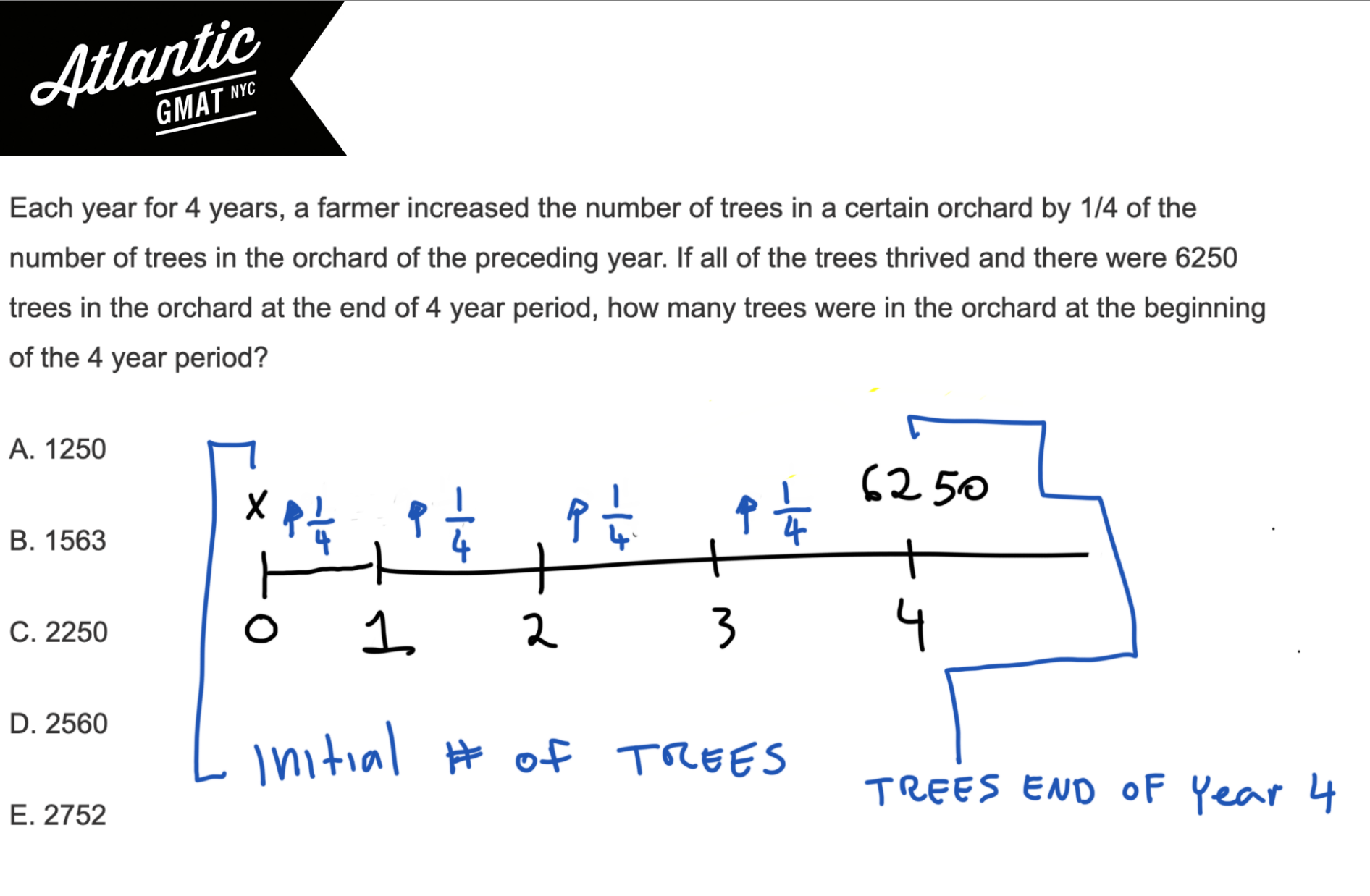Let’s call the first year x. How do you grow x by 1/4? Multiply x by (1 + 1/4) or 5/4. After the first year we have 5/4*x trees. Then we multiply that by 5/4 to get year 2. Multiply year 2 by 5/4 to get year 3. Multiple year 3 by 5/4 to get year 4. In the end you’ll have something equivalent to this for end of year 4: 5/4*5/4*5/4*5/4*x or (5/4)^4*x.

We know there were 6250 trees in the orchard at the end of the 4 year period so (5/4)^4*x = 6250. Now we need to simplify that.

Multiply by the reciprocal to isolate x. 6250*(4/5)^4.

5^4 = 625 and 6250 = 625*10 so cancel the 625.

That leaves 10 * 4^4.

Because of the 10 we know the answer must end in 0. Because of the 4^4 we know the answer must be a multiple of 4. That leaves only 2560, D. Divisibility can be very helpful for eliminating answer choices.

That said, 4^4 isn’t hard to calculate. It’s 2^8. Since 2^10 is 1024, 2^9 is 512, 2^8 is 256. I’d memorize 2^10 so you can work your way down to those higher powers of 2. It’s also good to know because it’s very close to 10^3 and powers of 10 come up a lot.

Here’s the full solution with diagram: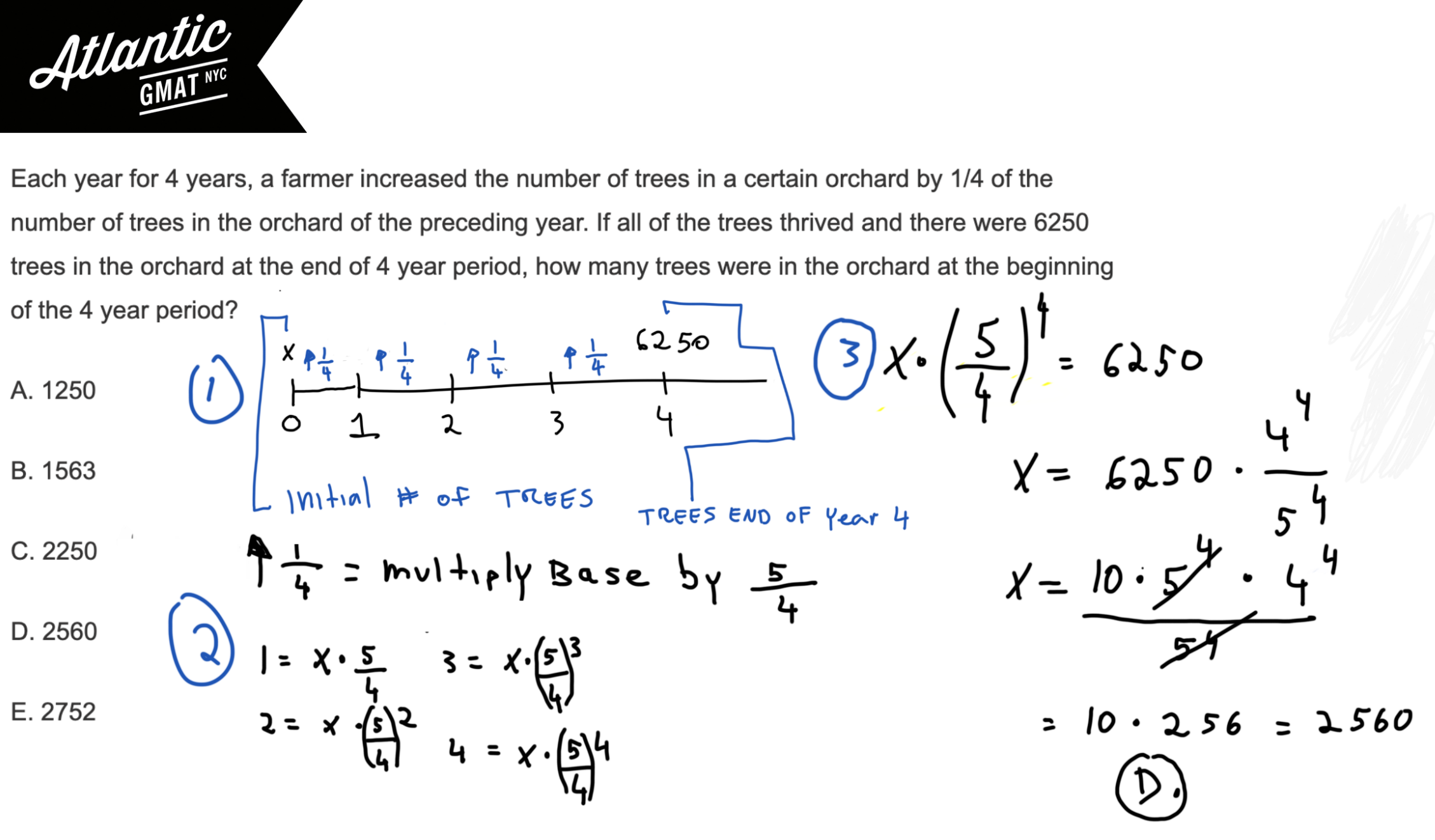Video Solution: Each year for 4 years, a farmer increased the number of trees in a certain orchard by 1/4 of the number of trees in the orchard of the preceding year. If all of the trees thrived and there were 6250 trees in the orchard at the end of the 4 year period, how many trees were in the orchard at the beginning of the 4 year period?

## Additional GMAT Problem Solving Practice Questions

Here’s a very similar one from the GMAT Prep Official Practice Tests 1 and 2: When a certain tree was first planted, it was 4 feet tall

# After driving to a riverfront parking lot, Bob plans to run south along the river, turn around, and return to the parking lot, running north along the same path. After running 3.25 miles south, he decides to run for only 50 minutes more. If Bob runs at a constant rate of 8 minutes per mile, how many miles farther south can he run and still be able to return to the parking lot in 50 minutes? GMAT Explanation, Video Solution, and More Practice Questions!

After driving to a riverfront parking lot, Bob plans to run south along the river, turn around, and return to the parking lot, running north along the same path. After running 3.25 miles south, he decides to run for only 50 minutes more. If Bob runs at a constant rate of 8 minutes per mile, how many miles farther south can he run and still be able to return to the parking lot in 50 minutes?

(A) 1.5

(B) 2.25

(C) 3.0

(D) 3.25

(E) 4.75

Here’s another work and rate example from the GMAT Official Guide. This one has very little follow through but can be challenging to set up. We see this one a lot in tutoring sessions. The mistake we see over and over again on this question and many others: rushing the reading and setup in favor of execution. There are a ton of GMAT questions that are mostly setup with very little execution (this one for instance). Setup will always help!

Ok, let’s go ahead and define the question: how many miles farther south can he run and still be able to return to the parking lot in 50 minutes?

So we want to figure the extra part of the run. We’ve got the initial journey (13/4 miles) then the extra (which we’re solving for so let’s call it “x”) and then the return trip which is x + 13/4 (3.25 miles). To the extent that you can make the thing you’re solving for the variable. Diagram below to make this clear.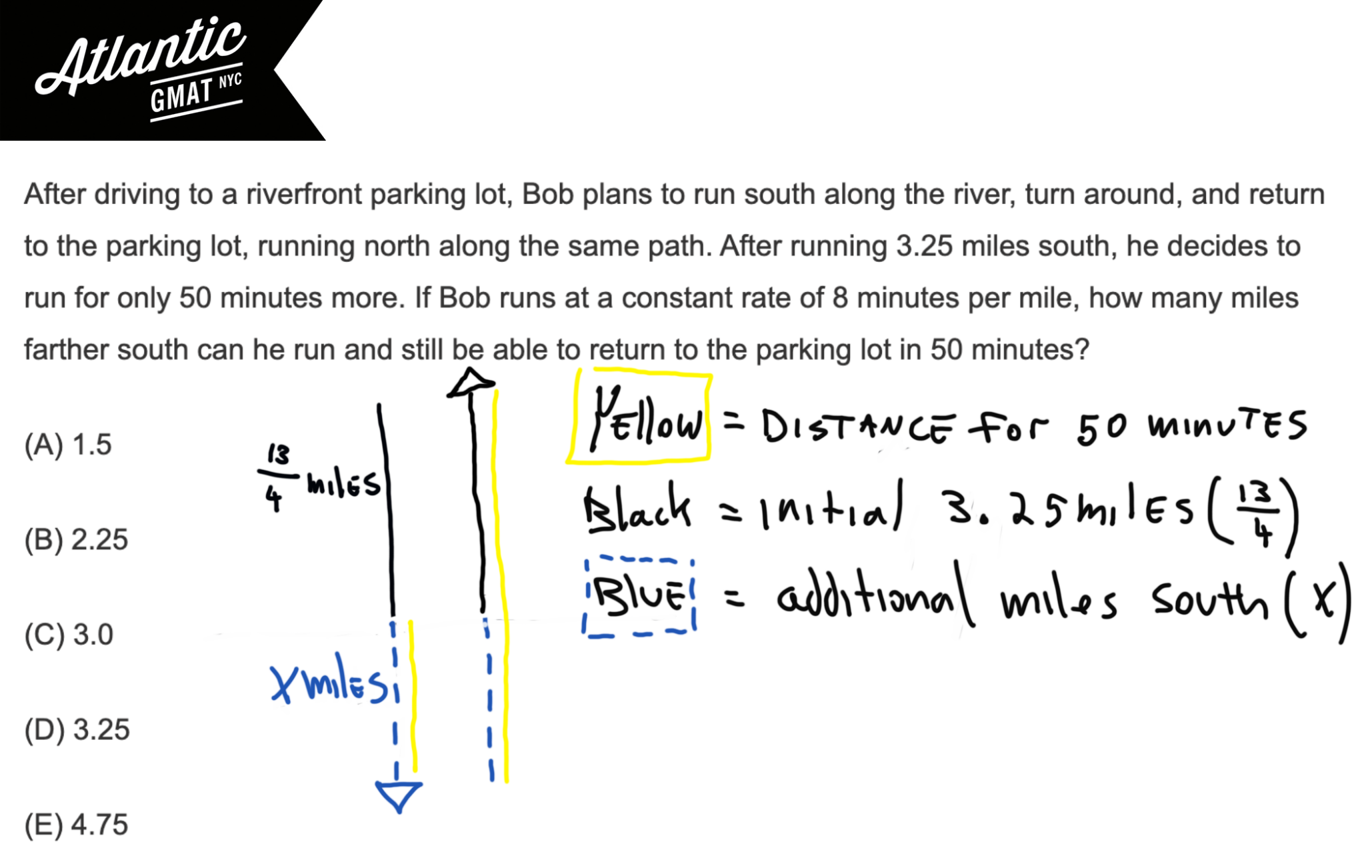Now we need to solve for the number of miles he can run while still getting back in 50 minutes. So set the time at 50 minutes. Set the rate at 1/8 (a constant rate of 8 minutes per mile). The distance is the trickiest part but once you’ve got the diagram shouldn’t be a problem. It’s the extra distance x, plus the extra distance back x, plus the original distance 13/4 (3.25 miles). So 2x + 13/4. That’s it. Pop that information into a rate T, solve for x, and you’re done!

50/8 = 2x + 13/4

50 = 16x + 26

16x = 24

x = 24/16

x = 1.5

How many miles farther south can he run and still be able to return to the parking lot in 50 minutes? 1.5 A.

Here’s the entire solution in a diagram: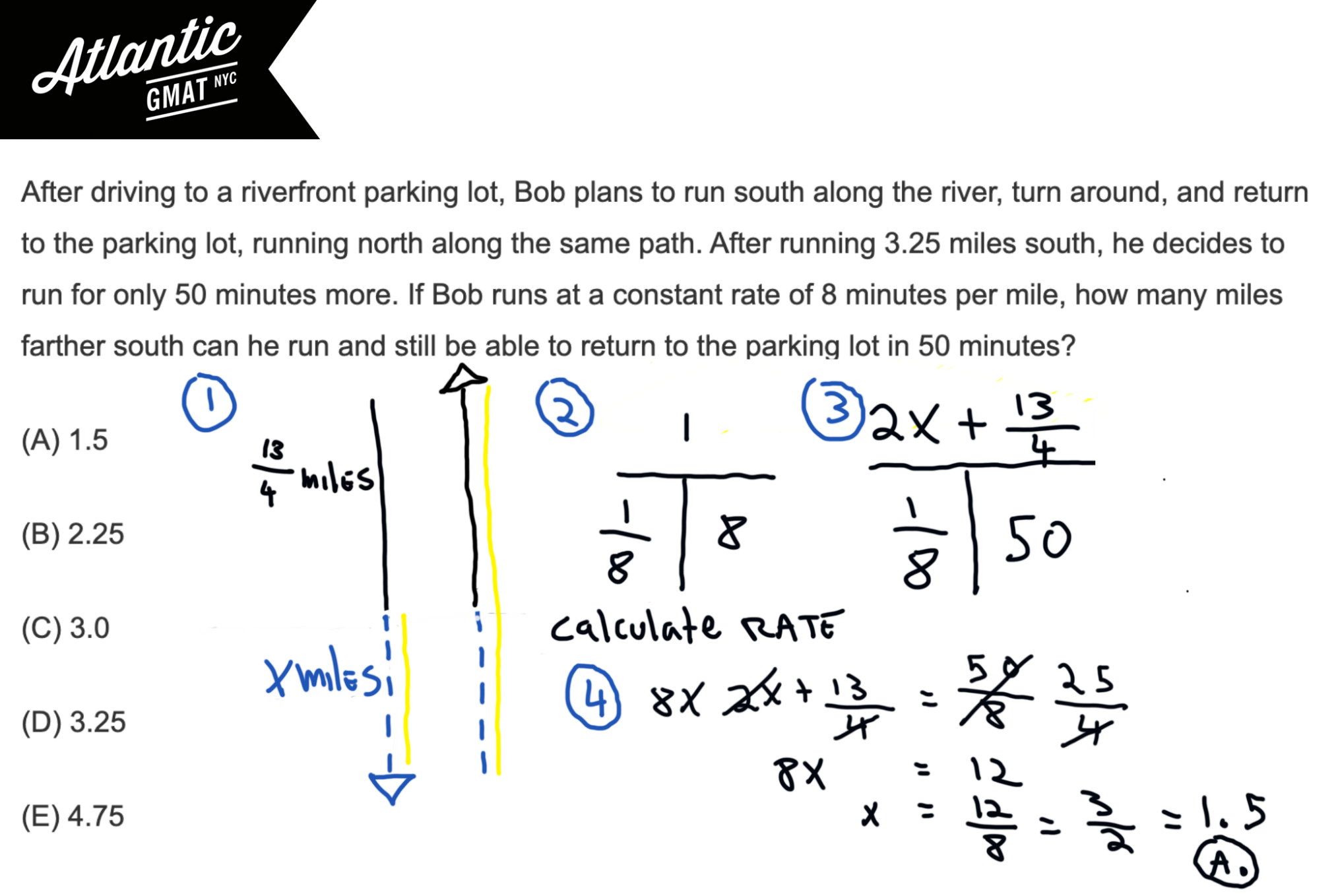## Additional GMAT Work and Rate Practice Questions

Here are another pair of challenging work and rate questions from the GMAT Official Guide on which you can practice setup at rate T’s:

During a trip, Francine traveled x percent of the total distance

Working simultaneously at their respective constant

And a very typical but challenging cooperative rate question from the GMAT Prep Tests:

Mary passed a certain gas station on a highway while traveling west at a constant speed of 50 miles per hour. Then, 15 minutes later, Paul passed the same gas station while traveling west at a constant speed of 60 miles per hour. If both drivers maintained their speeds and both remained on the highway for at least 2 hours, how long after he passed the gas station did Paul catch up with Mary?

And another three questions from GMAT Question of the day:

GMAT Question of the Day Distance, Work and Rate – Average Rate 1

GMAT Question of the Day Distance, Work and Rate – Average Rate 2

GMAT Question of the Day Distance, Work and Rate – Average Rate 3

# If Q is an odd number and the median of Q consecutive integers is 120, what is the largest of these integers? GMAT Explanation

If Q is an odd number and the median of Q consecutive integers is 120, what is the largest of these integers?

(A) (Q – 1)/2 + 120
(B) Q/2 + 119
(C) Q/2 + 120
(D) (Q + 119)/2
(E) (Q + 120)/2

To me this screams: pick a value for Q. Just make sure that you follow any rules outlined in the question. Always take your time to get things straight. That doesn’t mean sit and stare. Or obsessively re-read things. That means find the pace and level of detail that allows you to comprehend the question. Pause. Think. Digest.

## Let’s get to work!

So the median is 120, Q is an odd number, and there are Q consecutive integers. We’re looking for: the largest of these integers.

If 120 is the middle number (median) let’s just add one above and once below: 119 120 121

So, since Q represents the number of numbers, Q is 3. That value follows the rule: Q is odd.

## Plug back into the answer choices and look out for shortcuts (choices that clearly won’t match 121)

Now what? Now we do what we always do picking values and plug back into the answer choices. We need to sub in 3 for Q and output 121 (the largest of these integers).

You can rule out some of these without doing much since you can see fractions that clearly won’t work (B, C, E).

Then work out A and D.

D you can also eyeball. It’s way too small to equal 121.

It has to be A.

## Additional GMAT Statistics Example Questions!

Here’s a GMAT statistics puzzle (median/range) which will teach you how to work with a while bunch of unknowns. Also a good one to work on maximizing a value.

A set of 15 different integers has median of 25 and a range of 25. What is greatest possible integer that could be in this set?

Here’s a top shelf statistics, max/min question which we tackle in just about every GMAT preparation. it’s a great question to work on organizing a ton of variables. Also, it will help you understand how deal with a max/min scenario.

Seven pieces of rope have an average (arithmetic mean) length of 68 centimeters and a median length of 84 centimeters. If the length of the longest piece of rope is 14 centimeters more than 4 times the length of the shortest piece of rope, what is the maximum possible length, in centimeters, of the longest piece of rope?

Bread and butter GMAT statistics question. Not the most difficult but still very important. Be ready to interpret a chart/graph on test day. What isn’t challenging in practice can quickly become a swirling mess on GMAT day.

The table above shows the distribution of test scores for a group of management trainees. Which score interval contains the median of the 73 scores?

Great one to wrap your head around statistics concepts and how they can be tested on the GMAT. It’s a PS (problem solving) question but having this square will also help you on DS statistics questions.

Last month 15 homes were sold in Town X. The average (arithmetic mean) sale price of the homes was \$150,000 and the median sale price was \$130,000. Which of the following statements must be true?

Moderately challenging DS statistics range question from GMAT question of the day

And here’s a very challenging GMAT statistics range question from GMAT question of the day that requires algebra and number properties skills.

Good luck and happy studies!

# CONTACT

Atlantic GMAT Tutoring

405 East 51st St.

NY, NY 10022

(347) 669-3545